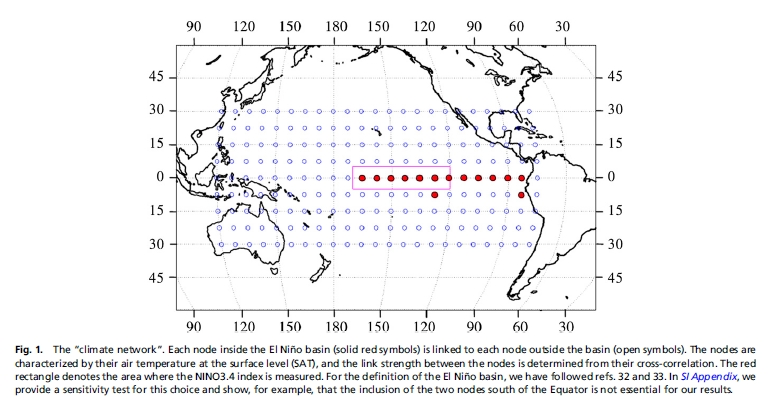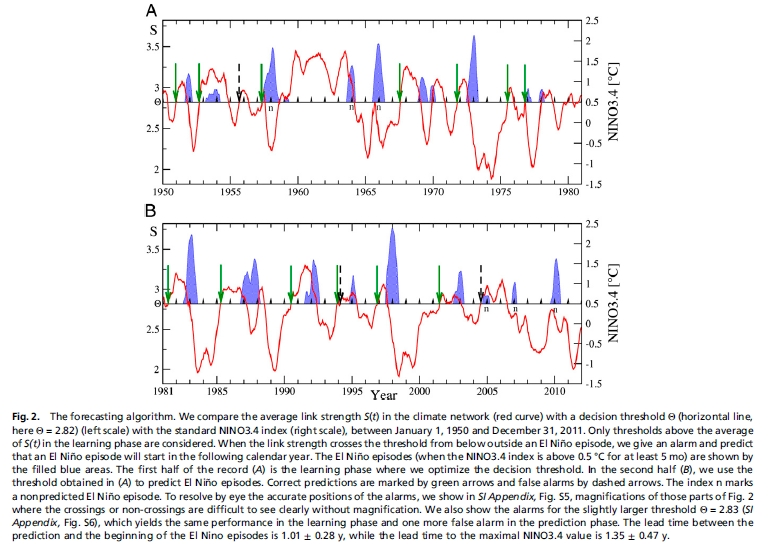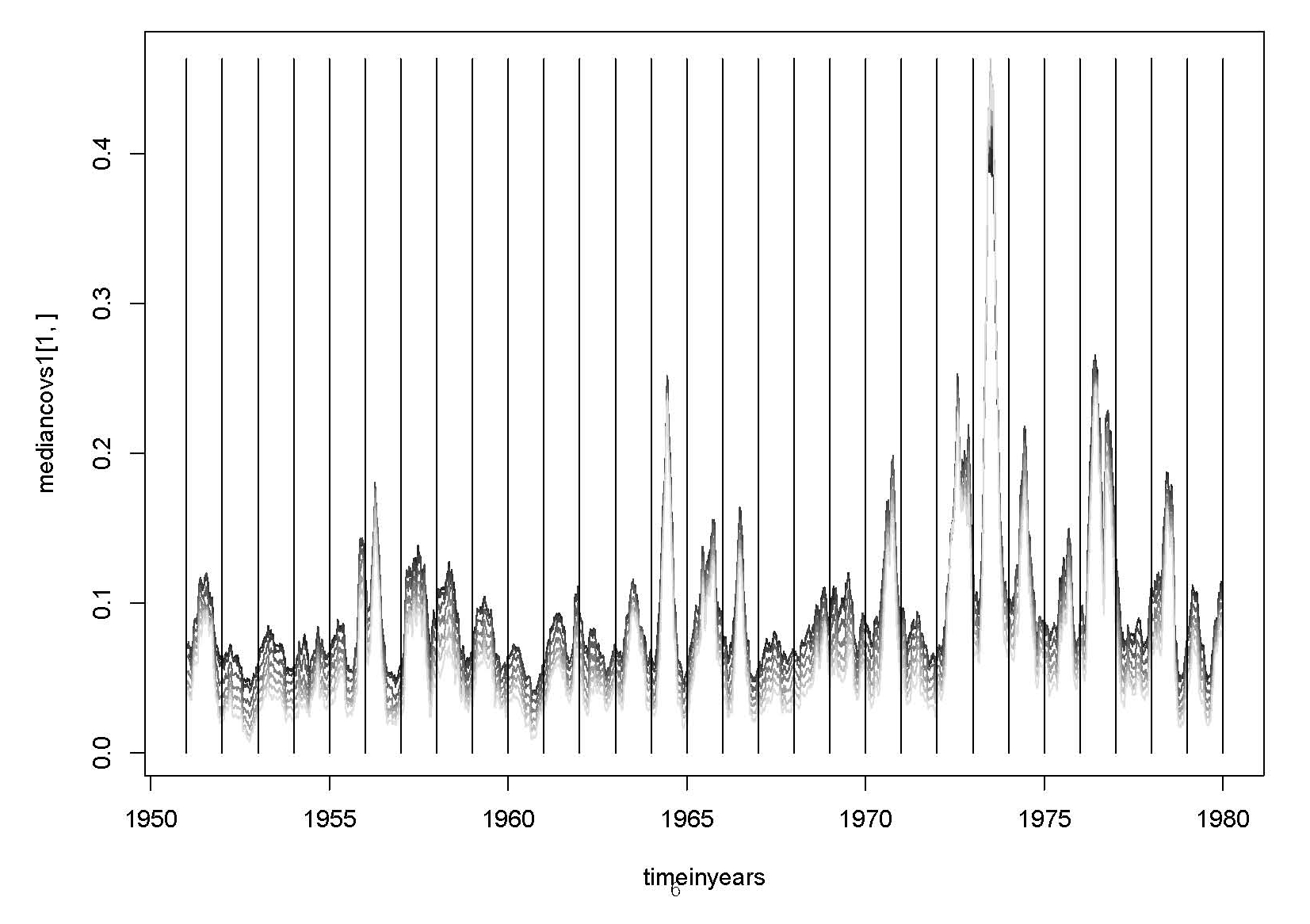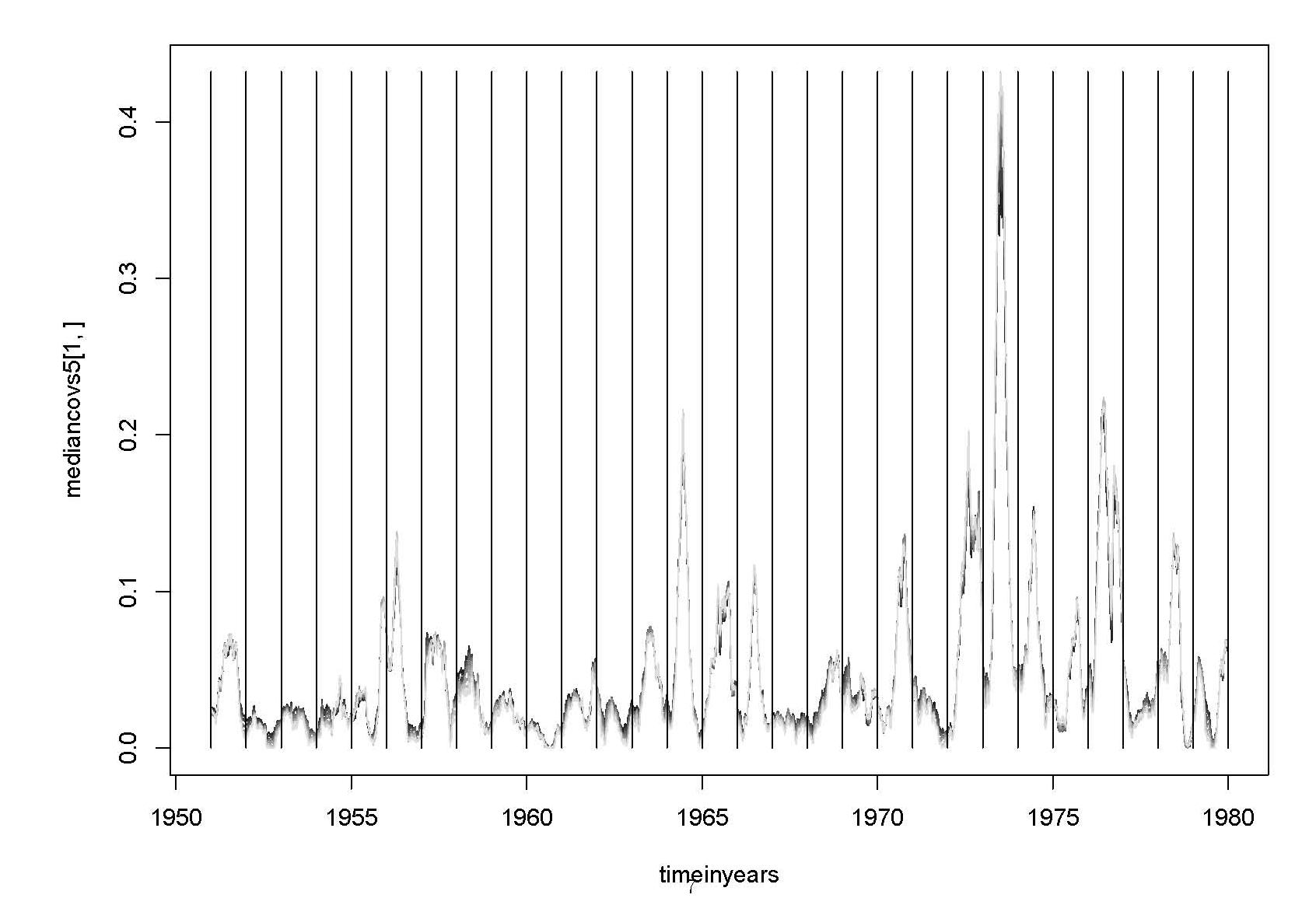# The Azimuth Project Experiments in El Niño analysis and prediction (Rev #24, changes)

Showing changes from revision #23 to #24: Added | Removed | Changed

# Contents

## Background

A short video explains El Niño issues in a simple way:

Tutorial information on climate networks, and their application to El Niño signal processing:

This paper explains how climate networks can be used to recognize when El Niño events are occurring:

This paper on El Niño prediction created a stir:

• Josef Ludescher, Avi Gozolchiani, Mikhail I. Bogachev, Armin Bunde, Shlomo Havlin, and Hans Joachim Schellnhuber, Very early warning of next El Niño, Proceedings of the National Academy of Sciences, February 2014. (Click title for free version, journal name for official version.)

The methodology is explained in this earlier paper:

• Josef Ludescher, Avi Gozolchiani, Mikhail I. Bogachev, Armin Bunde, Shlomo Havlin, and Hans Joachim Schellnhuber, Improved El Niño forecasting by cooperativity detection, Proceedings of the National Academy of Sciences, 30 May 2013. (For more discussion, go to the Azimuth Forum and also below.)

This paper is also relevant:

## Ludescher et al on El Niño forecasting by cooperativity detection

This paper:

uses data available from the National Centers for Environmental Prediction and the National Center for Atmospheric Research Reanalysis I Project:

More precisely, there’s a bunch of files here containing worldwide daily average temperatures on a 2.5° latitude × 2.5° longitude grid (144 × 73 grid points), from 1948 to 2010. If you go here the website will help you get data from within a chosen rectangle in a grid, for a chosen time interval. These are “netCDF files”; an R package for working with these files is here and some information on how they look is here.

The paper uses daily temperature data for “14 grid points in the El Niño basin and 193 grid points outside this domain” from 1981 to 2014, as shown here:That’s 207 locations and 34 years. The paper starts by taking these temperatures, computing the average temperature at each day of the year at each location, and subtracting this from the actual temperatures to obtain “temperature anomalies”. In other words, they use a big array of numbers like this: the temperature on March 21st 1990 at some location, minus the average temperature on all March 21sts from 1981 to 2014 at that location.

They process this data as explained here and attempt to use the result to predict the Nino3.4 index:

which is the area averaged sea surface temperature (SST) in the region 5°S-5°N and 170°-120°W.

Here is what they get:## Implementation

Our implementation is at Github

### Note on efficient implementation

The algorithm involves many calculations of time-delayed cross-covariances of the form

$C(m) = \frac{1}{N} \sum_{i=1}^N x_i y_{i+m} - \bigg( \frac{1}{N} \sum_{i=1}^N x_i \bigg) \bigg( \frac{1}{N} \sum_{i=1}^N y_{i+m} \bigg).$

Here $N=365$ is the period over which the covariances are found, and $m$ ranges over 0 to $M=200$. It can be re-arranged as

$C(m) = \frac{1}{N} \sum_{i=1}^N y_{i+m} d_i$

where

$d_i = x_i - \frac{1}{N} \sum_{i=1}^N x_i.$

The expression for $C(m)$ is now a convolution, so can be found efficiently using the discrete Fast Fourier transform.

## Visualizations of the data

### A first look at some of the data

This shows surface air temperatures over the Pacific for 1951:To see how this image was made: R code for pacific1951 image

### A second lookTo see how this image was made: R code to display 6 years of Pacific temperatures

### Third lookThe rectangle is roughly the area where the El Niño index NINO3.4 is defined.

### Conversion code

Here is an R script to convert netCDF data to a simple “flat” format which can be read by other programs. The output format is described in the code.

### Some correlations and covariances

The images below show local correlations and covariances of temperatures over the Pacific. Correlations are on the left and covariances on the right. They are calculated over the year of 1951. The correlations are shown on a scale where black is zero, white is 1. All of the values were positive; the smallest correlation was 0.26. The cube roots of the covariances are shown on a scale where black is zero and white is the maximum value. A linear mapping of values just shows a few pale pixels near the corners, with the rest black. Summary values for a set of covariances:

    Min.  1st Qu.   Median     Mean  3rd Qu.     Max.
0.09237  0.22520  0.40570  1.28000  1.11200 22.77000### More covariances

The PDF file Covariances near equator shows covariances between different places near the equator in the Pacific and at two different time delays. Most of the details are in the PDF, but some things are not:

• the first graph shows a 1-day delay and the second a 5-day delay.

• the graphs show the median of the covariances over the region

• black means zero geographical displacement, and paler greys show displacements increasing by 2.5 degrees.

The idea is to plot, for each 5 days from 1951 through 1979, for a region straddling the equator, and for 0 to 7 eastwards steps of 2.5 degrees, the covariances of the temperature over six months (183 days). Here are the results:### Covariance maps 1951-1979The image shows one map of the Pacific for each quarter for the years 1951 through 1979. On the right, the NINO3.4 index is shown for the year.

The area is that used by Ludescher et al (2013). The “El Nino basin”, as defined by Ludescher et al (2013) is the black region along the Equator towards the East, plus two pixels below. For every other pixel i, the sum TC(i) of the covariances between i and the 14 pixels in the basin is shown. The covariances are calculated over the previous year. The absolute values are “squashed” by before conversion to colours. Negative values of TC(i) are red, positive values green, paler meaning bigger in absolute value.
Very big values are shown by bright red and green.

#### More detail

The climatological seasonal cycle (mean over years for each grid point, each day-in-year) is subtracted. The data is spatially subsampled into 7.5 by 7.5 degree squares. There are 9 by 23 such squares. The covariances are calculated for a day in the middle of each quarter. The covariances are calculated over a period of 365 days. There is no time delay between the periods. The TC(i) values are squashed by sign(TC(i)) * sqrt(abs(TC(i))) before conversion to colours. The range -3,3 is mapped to dull shades; below -3 is bright red, above 3 bright green.

The El Nino index is from http://www.cpc.ncep.noaa.gov/products/analysis_monitoring/ensostuff/detrend.nino34.ascii.txt.

To see how this image was made: R code to make covariance maps 1951-1979

### Studying the dependence of covariance on distance

The graphs below are based on David Tanzer’s numbers in this thread in the forum. The top three graphs show the square roots of the absolute values of the covariances. The bottom three are similar, but the values are scaled so that the covariance for the smallest distance is always 1.### First attempt at replicating Ludescher et al

First attempt at replicating Ludescher et al 2013. It uses time-delays of up to 295 (not 200). It does every 73rd day (not every 10). The result appears similar to Fig 2 in their paper (see image above in section “Ludescher et al on El Niño forecasting by cooperativity detection”) but with lower values of S(t).For dataset source:

• Kalnay et al.,The NCEP/NCAR 40-year reanalysis project, Bull. Amer. Meteor. Soc., 77, 437-470, 1996.

category: climate, experiments